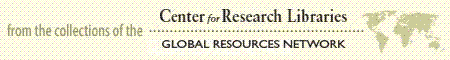Document Preview
• Full Text
• Scholarly Journal

# ON THE MENSTRUAL WAVE: METHOD OF ANALYSIS CASE A.--Average curve of three Menstrual Periods of 23 days each Average temperature =99.31° CASE B--Average curve of two Menstrual Periods of 30 days Average temperature =99.66° CASE C.--Averages of One Month only. Average temperature =99.46° CASE D.--Average of two waves of 32 days each. Average temp. =99.20°